Share

Books Shortlist

# NCERT solutions for Class 10 Mathematics chapter 5 - Arithmetic Progressions

## Mathematics Textbook for Class 10

#### NCERT Mathematics Class 10## Chapter 5: Arithmetic Progressions

Ex. 5.10Ex. 5.20Ex. 5.30OthersEx. 5.40

#### Chapter 5: Arithmetic Progressions Exercise 5.10 solutions [Pages 99 - 100]

Ex. 5.10 | Q 1.1 | Page 99

In which of the following situations, does the list of numbers involved make as arithmetic progression and why?

The taxi fare after each km when the fare is Rs 15 for the first km and Rs 8 for each additional km

Ex. 5.10 | Q 1.2 | Page 99

In which of the following situations, does the list of numbers involved make an arithmetic progression and why?

The amount of air present in a cylinder when a vacuum pump removes 1/4 of the air remaining in the cylinder at a time.

Ex. 5.10 | Q 1.3 | Page 99

In which of the following situations, does the list of numbers involved make as arithmetic progression and why?

The cost of digging a well after every metre of digging, when it costs Rs 150 for the first metre and rises by Rs 50 for each subsequent metre.

Ex. 5.10 | Q 1.4 | Page 99

In which of the following situations, does the list of numbers involved make as arithmetic progression and why?

The amount of money in the account every year, when Rs 10000 is deposited at compound interest at 8 % per annum.

Ex. 5.10 | Q 2.1 | Page 99

Write first four terms of the A.P. when the first term a and the common differenced are given as follows a = 10, d = 10

Ex. 5.10 | Q 2.2 | Page 99

Write first four terms of the A.P. when the first term a and the common differenced are given as follows a = -2, d = 0

Ex. 5.10 | Q 2.3 | Page 99

Write first four terms of the A.P. when the first term a and the common differenced are given as follows a = 4, d = - 3

Ex. 5.10 | Q 2.4 | Page 99

Write first four terms of the A.P. when the first term a and the common differenced are given as follows a = -1, d = 1/2

Ex. 5.10 | Q 2.5 | Page 99

Write first four terms of the A.P. when the first term a and the common differenced are given as follows a = - 1.25, d = - 0.25

Ex. 5.10 | Q 3.1 | Page 99

For the following APs, write the first term and the common difference 3, 1, – 1, – 3, . . .

Ex. 5.10 | Q 3.2 | Page 99

For the following A.P.s, write the first term and the common difference -5, - 1, 3, 7

Ex. 5.10 | Q 3.3 | Page 99

For the following A.P.s, write the first term and the common difference 1/3, 5/3, 9/3, 13/3 ....

Ex. 5.10 | Q 3.4 | Page 99

For the following A.P.s, write the first term and the common difference. 0.6, 1.7, 2.8, 3.9

Ex. 5.10 | Q 4.01 | Page 99

Which of the following are APs? If they form an A.P. find the common difference d and write three more terms 2, 4, 8, 16 …

Ex. 5.10 | Q 4.02 | Page 99

Which of the following are APs? If they form an A.P. find the common difference d and write three more terms 2, 5/2, 3, 7/2 ....

Ex. 5.10 | Q 4.03 | Page 99

Which of the following are APs? If they form an A.P. find the common difference d and write three more terms. -1.2, -3.2, -5.2, -7.2 …

Ex. 5.10 | Q 4.04 | Page 99

Which of the following are APs? If they form an A.P. find the common difference d and write three more terms. -10, - 6, - 2, 2 …

Ex. 5.10 | Q 4.05 | Page 99

Which of the following are APs? If they form an A.P. find the common difference d and write three more terms. 3, 3 + sqrt2, 3 + 2sqrt2, 3 + 3sqrt2

Ex. 5.10 | Q 4.06 | Page 99

Which of the following are APs? If they form an A.P. find the common difference d and write three more terms. 0.2, 0.22, 0.222, 0.2222 ….

Ex. 5.10 | Q 4.07 | Page 99

Which of the following are APs? If they form an A.P. find the common difference d and write three more terms. 0, - 4, - 8, - 12 …

Ex. 5.10 | Q 4.08 | Page 99

Which of the following are APs? If they form an A.P. find the common difference d and write three more terms. -1/2, -1/2, -1/2, -1/2 ....

Ex. 5.10 | Q 4.09 | Page 100

Which of the following are APs? If they form an A.P. find the common difference d and write three more terms 1, 3, 9, 27 …

Ex. 5.10 | Q 4.1 | Page 100

Which of the following are APs? If they form an A.P. find the common difference d and write three more terms. a, 2a, 3a, 4a …

Ex. 5.10 | Q 4.11 | Page 100

Which of the following are APs? If they form an A.P. find the common difference d and write three more terms a, a2, a3, a4 …

Ex. 5.10 | Q 4.12 | Page 100

Which of the following are APs? If they form an A.P. find the common difference d and write three more terms. sqrt2, sqrt8, sqrt18, sqrt32 ...

Ex. 5.10 | Q 4.13 | Page 100

Which of the following are APs? If they form an A.P. find the common difference d and write three more terms sqrt3, sqrt6, sqrt9, sqrt12 ...

Ex. 5.10 | Q 4.14 | Page 100

Which of the following are APs? If they form an A.P. find the common difference d and write three more terms. 12, 32, 52, 72 …

Ex. 5.10 | Q 4.15 | Page 100

Which of the following are APs? If they form an A.P. find the common difference d and write three more terms. 12, 52, 72, 73 …

#### Chapter 5: Arithmetic Progressions Exercise 5.20 solutions [Pages 105 - 107]

Ex. 5.20 | Q 1 | Page 105

Fill in the blanks in the following table, given that a is the first term, d the common difference and an the nth term of the AP: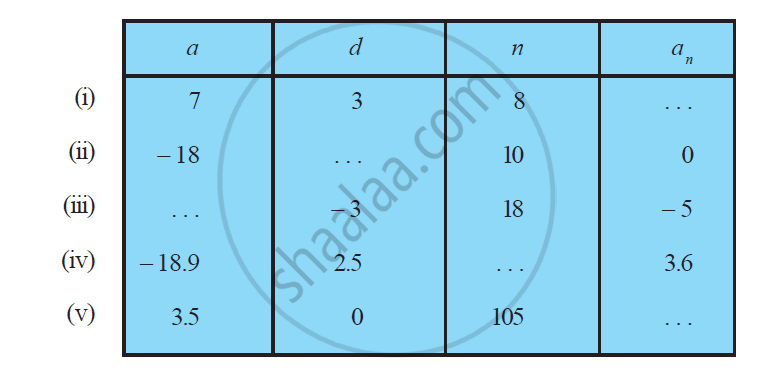Ex. 5.20 | Q 2.1 | Page 106

Choose the correct choice in the following and justify 30th term of the AP: 10, 7, 4, . . . , is

• 97

•  77

• –77

• – 87

Ex. 5.20 | Q 2.2 | Page 106

Choose the correct choice in the following and justify

11th term of the A.P. -3, -1/2, 2 .... is

• 28

• 22

• –38

• – 481/2

Ex. 5.20 | Q 3.1 | Page 106

In the following APs, find the missing terms in the boxes :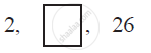Ex. 5.20 | Q 3.2 | Page 106

In the following APs find the missing term in the boxes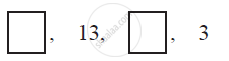Ex. 5.20 | Q 3.3 | Page 106

In the following APs, find the missing terms in the boxes :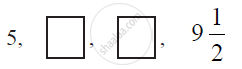Ex. 5.20 | Q 3.4 | Page 106

In the following APs, find the missing terms in the boxes :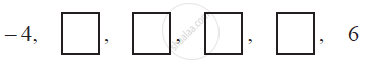Ex. 5.20 | Q 3.5 | Page 106

In the following APs, find the missing terms in the boxes :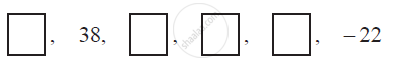Ex. 5.20 | Q 4 | Page 106

Which term of the AP : 3, 8, 13, 18, . . . ,is 78?

Ex. 5.20 | Q 5.1 | Page 106

Find the number of terms in the following A.P. : 7, 13, 19, . . . , 205

Ex. 5.20 | Q 5.2 | Page 106

Find the number of terms in each of the following A.P. 18,15 1/2, 13, . . . , – 47

Ex. 5.20 | Q 6 | Page 106
Check whether -150 is a term of the A.P. 11, 8, 5, 2, …
Ex. 5.20 | Q 7 | Page 106

Find the 31st term of an A.P. whose 11th term is 38 and the 16th term is 73

Ex. 5.20 | Q 8 | Page 106

An A.P. consists of 50 terms of which 3rd term is 12 and the last term is 106. Find the 29th term

Ex. 5.20 | Q 9 | Page 106

If the 3rd and the 9th terms of an AP are 4 and – 8 respectively, which term of this AP is zero?

Ex. 5.20 | Q 10 | Page 106

The 17th term of an AP exceeds its 10th term by 7. Find the common difference.

Ex. 5.20 | Q 11 | Page 106

Which term of the A.P. 3, 15, 27, 39, … will be 132 more than its 54thterm?

Ex. 5.20 | Q 12 | Page 106

Two APs have the same common difference. The difference between their 100th term is 100, what is the difference between their 1000thterms?

Ex. 5.20 | Q 13 | Page 106

How many three digit numbers are divisible by 7

Ex. 5.20 | Q 14 | Page 106

How many multiples of 4 lie between 10 and 250?

Ex. 5.20 | Q 15 | Page 106

For what value of n, are the nth terms of two APs 63, 65, 67, and 3, 10, 17, … equal?

Ex. 5.20 | Q 16 | Page 106

Determine the A.P. whose third term is 16 and the 7th term exceeds the 5th term by 12.

Ex. 5.20 | Q 17 | Page 107

Find the 20th term from the last term of the A.P. 3, 8, 13, …, 253

Ex. 5.20 | Q 18 | Page 107

The sum of 4th and 8th terms of an A.P. is 24 and the sum of the 6th and 10th terms is 44. Find the first three terms of the A.P.

Ex. 5.20 | Q 19 | Page 107

Subba Rao started work in 1995 at an annual salary of Rs 5000 and received an increment of Rs 200 each year. In which year did his income reach Rs 7000?

Ex. 5.20 | Q 20 | Page 107

Ramkali saved Rs 5 in the first week of a year and then increased her weekly saving by Rs 1.75. If in the nthweek, her week, her weekly savings become Rs 20.75, find n.

#### Chapter 5: Arithmetic Progressions Exercise 5.30 solutions [Pages 112 - 114]

Ex. 5.30 | Q 1.1 | Page 112

Find the sum of the following APs:

2, 7, 12, . . ., to 10 terms.

Ex. 5.30 | Q 1.2 | Page 112

Find the sum of the following APs.

− 37, − 33, − 29 ,…, to 12 terms

Ex. 5.30 | Q 1.3 | Page 112

Find the sum of the following APs.

0.6, 1.7, 2.8 ,…….., to 100 terms

Ex. 5.30 | Q 1.4 | Page 112

Find the sum of the following APs. 1/15, 1/12, 1/10 , ...... , to 11 terms

Ex. 5.30 | Q 2.1 | Page 112

Find the sums given below : 7 + 10 1/2 + 14 + .................. +84

Ex. 5.30 | Q 2.2 | Page 112

Find the sums given below :

34 + 32 + 30 + . . . + 10

Ex. 5.30 | Q 2.3 | Page 112

Find the sums given below :

–5 + (–8) + (–11) + . . . + (–230)

Ex. 5.30 | Q 3.01 | Page 112

In an AP:

Given a = 5, d = 3, an = 50, find n and Sn.

Ex. 5.30 | Q 3.02 | Page 112

In an AP

Given a = 7, a13 = 35, find d and S13.

Ex. 5.30 | Q 3.03 | Page 112

In an AP

Given a12 = 37, d = 3, find a and S12.

Ex. 5.30 | Q 3.04 | Page 112

In an AP

Given a3 = 15, S10 = 125, find d and a10.

Ex. 5.30 | Q 3.05 | Page 112

In an AP

Given d = 5, S9 = 75, find a and a9.

Ex. 5.30 | Q 3.06 | Page 112

In an AP:

Given a = 2, d = 8, Sn = 90, find and an.

Ex. 5.30 | Q 3.07 | Page 112

In an AP

Given a = 8, an = 62, Sn = 210, find n and d.

Ex. 5.30 | Q 3.08 | Page 112

In an AP .Given an = 4, d = 2, Sn = − 14, find n and a.

Ex. 5.30 | Q 3.09 | Page 112

In an AP

Given a = 3, n = 8, S = 192, find d.

Ex. 5.30 | Q 3.1 | Page 112

In an AP:

Given l = 28, S = 144, and there are total 9 terms. Find a.

Ex. 5.30 | Q 4 | Page 113

How many terms of the AP. 9, 17, 25 … must be taken to give a sum of 636?

Ex. 5.30 | Q 5 | Page 113

The first term of an AP is 5, the last term is 45 and the sum is 400. Find the number of terms and the common difference.

Ex. 5.30 | Q 6 | Page 113

The first and the last term of an AP are 17 and 350 respectively. If the common difference is 9, how many terms are there and what is their sum?

Ex. 5.30 | Q 7 | Page 113

Find the sum of first 22 terms of an AP in which d = 7 and 22nd term is 149.

Ex. 5.30 | Q 8 | Page 113

Find the sum of first 51 terms of an AP whose second and third terms are 14 and 18 respectively.

Q 9 | Page 113

If the sum of first 7 terms of an A.P. is 49 and that of its first 17 terms is 289, find the sum of first n terms of the A.P.

Ex. 5.30 | Q 10.1 | Page 113

Show that a1, a… , an , … form an AP where an is defined as below

an = 3 + 4n

Also find the sum of the first 15 terms.

Ex. 5.30 | Q 10.2 | Page 113

Show that a1, a… , an , … form an AP where an is defined as below

an = 9 − 5n

Also find the sum of the first 15 terms.

Ex. 5.30 | Q 11 | Page 113

If the sum of the first n terms of an AP is 4n − n2, what is the first term (that is S1)? What is the sum of first two terms? What is the second term? Similarly find the 3rd, the10th and the nth terms.

Ex. 5.30 | Q 12 | Page 113

Find the sum of first 40 positive integers divisible by 6.

Ex. 5.30 | Q 13 | Page 113

Find the sum of first 15 multiples of 8.

Ex. 5.30 | Q 14 | Page 113

Find the sum of the odd numbers between 0 and 50.

Ex. 5.30 | Q 15 | Page 113

A contract on construction job specifies a penalty for delay of completion beyond a certain date as follows: Rs. 200 for the first day, Rs. 250 for the second day, Rs. 300 for the third day, etc., the penalty for each succeeding day being Rs. 50 more than for the preceding day. How much money the contractor has to pay as penalty, if he has delayed the work by 30 days.

Ex. 5.30 | Q 16 | Page 113

A sum of Rs 700 is to be used to give seven cash prizes to students of a school for their overall academic performance. If each prize is Rs 20 less than its preceding prize, find the value of each of the prizes.

Ex. 5.30 | Q 17 | Page 113

In a school, students thought of planting trees in and around the school to reduce air pollution. It was decided that the number of trees, that each section of each class will plant, will be the same as the class, in which they are studying, e.g., a section of class I will plant 1 tree, a section of class II will plant 2 trees and so on till class XII. There are three sections of each class. How many trees will be planted by the students?

Ex. 5.30 | Q 18 | Page 113

A spiral is made up of successive semicircles, with centres alternately at A and B, starting with centre at A of radii 0.5, 1.0 cm, 1.5 cm, 2.0 cm, ……… as shown in figure. What is the total length of such a spiral made up of thirteen consecutive semicircles? (Take pi = 22/7 )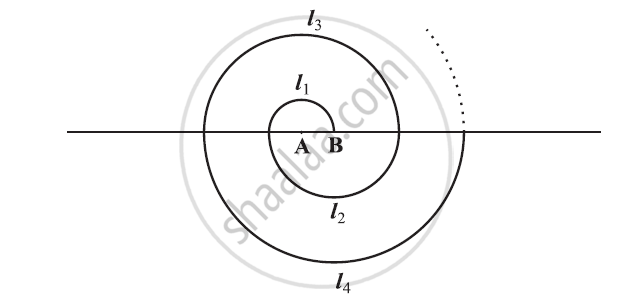Ex. 5.30 | Q 19 | Page 114

200 logs are stacked in the following manner: 20 logs in the bottom row, 19 in the next row, 18 in the row next to it and so on. In how many rows are the 200 logs placed and how many logs are in the top row?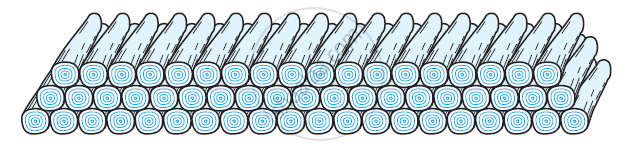Ex. 5.30 | Q 20 | Page 114

In a potato race, a bucket is placed at the starting point, which is 5 m from the first potato and other potatoes are placed 3 m apart in a straight line. There are ten potatoes in the line.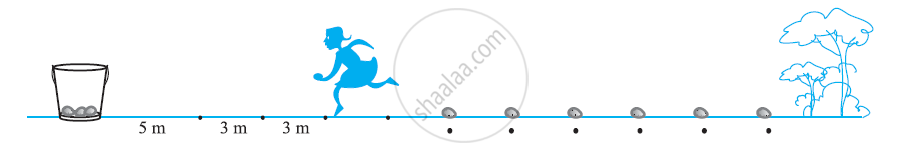A competitor starts from the bucket, picks up the nearest potato, runs back with it, drops it in the bucket, runs back to pick up the next potato, runs to the bucket to drop it in, and she continues in the same way until all the potatoes are in the bucket. What is the total distance the competitor has to run?

[Hint: to pick up the first potato and the second potato, the total distance (in metres) run by a competitor is 2 × 5 + 2 ×(5 + 3)]

#### Chapter 5: Arithmetic Progressions Exercise 5.40 solutions [Page 115]

Ex. 5.40 | Q 1 | Page 115

Which term of the A.P. 121, 117, 113 … is its first negative term? [Hint: Find n for an < 0]

Ex. 5.40 | Q 2 | Page 115

The sum of the third and the seventh terms of an AP is 6 and their product is 8. Find the sum of first sixteen terms of the AP.

Ex. 5.40 | Q 3 | Page 115

A ladder has rungs 25 cm apart. (See figure). The rungs decrease uniformly in length from 45 cm at the bottom to 25 cm at the top. If the top and bottom rungs are 2 1/2 m apart, what is the length of the wood required for the rungs?

[Hint: number of rungs = 250/25 ]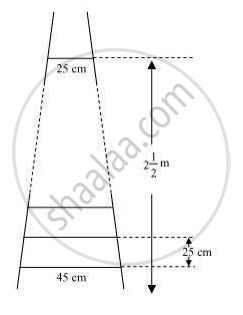Ex. 5.40 | Q 4 | Page 115

The houses in a row numbered consecutively from 1 to 49. Show that there exists a value of X such that sum of numbers of houses preceding the house numbered X is equal to sum of the numbers of houses following X.

Ex. 5.40 | Q 5 | Page 115

A small terrace at a football ground comprises of 15 steps each of which is 50 m long and built of solid concrete. Each step has a rise of 1/4 m and a tread of 1/2 m (See figure) calculate the total volume of concrete required to build the terrace.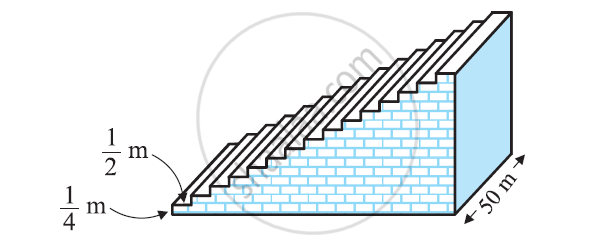[Hint : Volume of concrete required to build the first step = 1/4 x 1/2 x 50m3]

## Chapter 5: Arithmetic Progressions

Ex. 5.10Ex. 5.20Ex. 5.30OthersEx. 5.40

#### NCERT Mathematics Class 10## NCERT solutions for Class 10 Mathematics chapter 5 - Arithmetic Progressions

NCERT solutions for Class 10 Maths chapter 5 (Arithmetic Progressions) include all questions with solution and detail explanation. This will clear students doubts about any question and improve application skills while preparing for board exams. The detailed, step-by-step solutions will help you understand the concepts better and clear your confusions, if any. Shaalaa.com has the CBSE Mathematics Textbook for Class 10 solutions in a manner that help students grasp basic concepts better and faster.

Further, we at shaalaa.com are providing such solutions so that students can prepare for written exams. NCERT textbook solutions can be a core help for self-study and acts as a perfect self-help guidance for students.

Concepts covered in Class 10 Mathematics chapter 5 Arithmetic Progressions are General Term of an Arithmetic Progression, nth Term of an AP, Sum of First n Terms of an AP, Arithmetic Progression, Derivation of the n th Term, Application in Solving Daily Life Problems, Arithmetic Progressions Examples and Solutions.

Using NCERT Class 10 solutions Arithmetic Progressions exercise by students are an easy way to prepare for the exams, as they involve solutions arranged chapter-wise also page wise. The questions involved in NCERT Solutions are important questions that can be asked in the final exam. Maximum students of CBSE Class 10 prefer NCERT Textbook Solutions to score more in exam.

Get the free view of chapter 5 Arithmetic Progressions Class 10 extra questions for Maths and can use shaalaa.com to keep it handy for your exam preparation

S